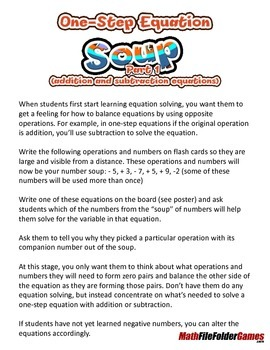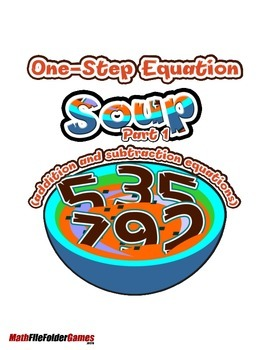# Solving Equations - One-Step Equation Soup

Rated 5 out of 5, based on 1 reviews
1 Rating;
6th - 9th, Homeschool
Subjects
Resource Type
Formats Included
• Zip
Report this resource to TPT

### Description

Solving Equations - One-Step Equation Soup, Part 1 (addition and subtraction equations) & Part 2 (multiplication and division)

When students first start learning equation solving, you want them to get a feeling for how to balance equations by using opposite operations. For example, in one-step equations if the original operation is addition, you’ll use subtraction to solve the equation.

Write the following operations....

=============================================
This solving equations activity is also included in my Focus On Solving Equations BUNDLE. This bundle comes with 31 solving equations activities, click here to save!
=============================================

Total Pages